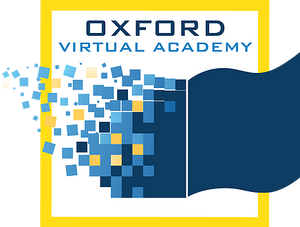# Math 6 RightStart Math - (Hybrid)

Grade 6 uses hands-on and visually using a geometric approach. With a drawing board, T-square, triangles, compass, and goniometer, the student explores fractions, area, ratios, angles, Pythagorean theorem, and square roots. Circles, pi, arcs, along with reflections, rotations, and symmetry are taught all the while practicing arithmetic, fractions, and decimals. Some algebraic concepts are introduced. The history of mathematics is woven throughout the lessons.

* Prerequisites: None

* This course includes an optional learning experience.